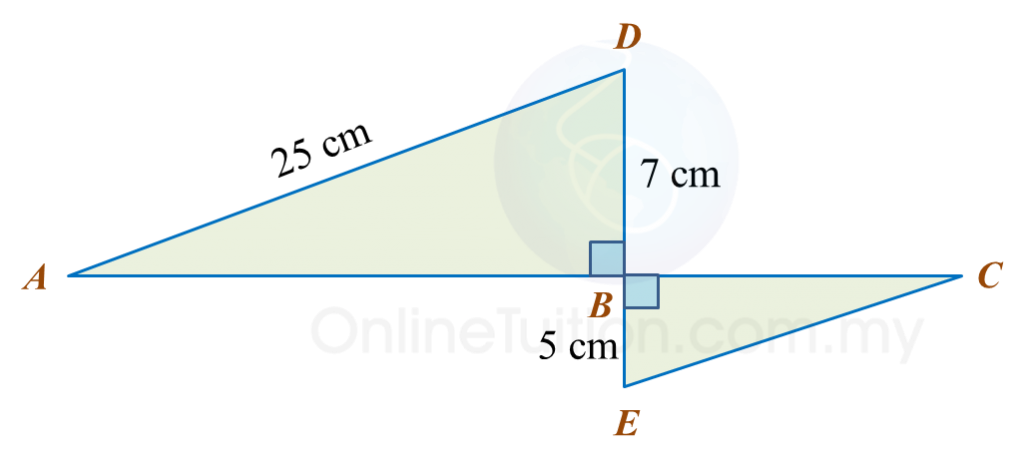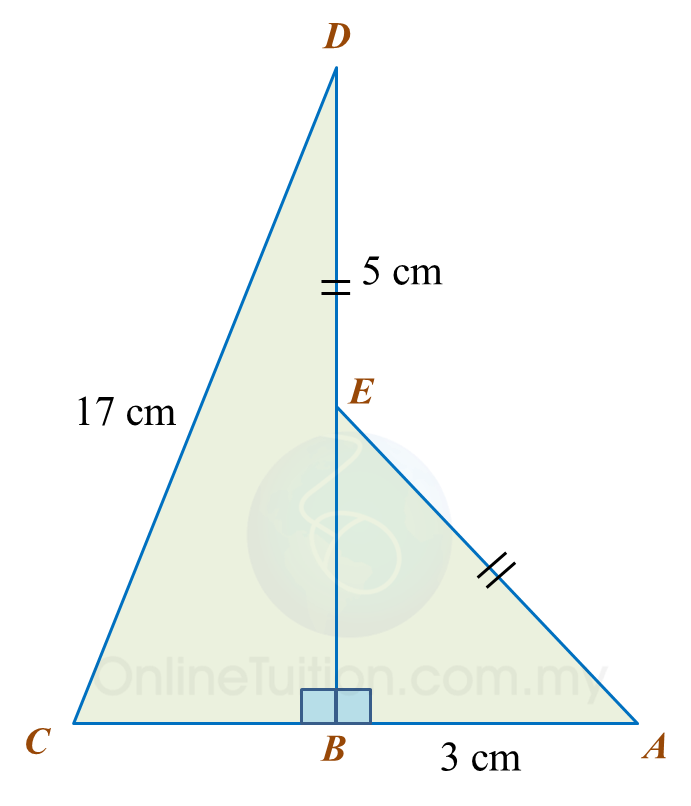# 6.2.2 Pythagoras’ Theorem, PT3 Focus Practice

Question 6:
In diagram below, ABCH is a square and DEFG is a rectangle. HCDE is a straight line and HC = CD.Find the perimeter, in cm, of the whole diagram.

Solution:

Question 7:
In the diagram, ABC and EFD are right-angled triangles.Calculate the perimeter, in cm, of the shaded region.

Solution:

Question 8:
In the diagram, ABD and BCE are right-angled triangles. ABC and DBE are straight lines. The length of AB is twice the length of BC.Calculate the length, in cm, of CE.

Solution:

Question 9:
Diagram below shows two right-angled triangles, ABE and CBD. BED is a straight line.Find the length, in cm, of BC. Round off the answer to two decimal places.

Solution:

Question 10:
Diagram below shows two right-angled triangles, ABC and ADE. ACD is a straight line.Find the length, in cm, of AE. Round off the answer to one decimal places.

Solution:

# 6.2.1 Pythagoras’ Theorem, PT3 Focus Practice

6.2.1 Pythagoras’ Theorem, PT3 Focus Practice

Question 1:
In the diagram, ABC is a right-angled triangle and BCD is a straight line. Calculate the length of AD, correct to two decimal places.

Solution:
In ∆ ABC,
BC= 52 – 42
= 25 – 16
= 9
BC = √9
= 3 cm

In ∆ ABD,
BD = BC + CD
= 3 + 6
= 9
= 16 + 81
= 97
= 9.849
= 9.85 cm

Question 2:
In the diagram shows two right-angled triangles. Calculate the perimeter of the whole diagram.

Solution:
In ∆ ABC,
AC= 62 + 82
= 36 + 64
= 100
AC = √100
= 10 cm

In ∆ EDC,
EC= 122 + 52
= 144 + 25
= 169
EC = √169
= 13 cm

Perimeter of the whole diagram
= AB + BC + CE + DE + AD
= 6 + 8 + 13 + 12 + 5
= 44 cm

Question 3:
Diagram below shows a triangle ACD and ABC is a straight line.
Calculate the length, in cm, of AD.

Solution:
In ∆ DBC,
BC= 252 – 242
= 625 – 576
= 49
BC = √49
= 7 cm
AB = 17 – 7 = 10 cm

In ∆ DAB,
= 100 + 576
= 676
= 26 cm

Question 4:
In diagram below ABDE is a square and EDC is a straight line.
The area of the square ABDE is 144 cm2.
Calculate the length, in cm, of BC.

Solution:
BD = √144
= 12 cm
BC= 122 + 92
= 144 + 81
= 225
BC = √225
= 15 cm

Question 5:
In the diagram, ABCD is a trapezium and AED is a right-angled triangle.

Calculate the area, in cm2, of the shaded region.

Solution:
= 25 + 144
= 169
= 13 cm
Area of trapezium ABDC
= ½ (13 + 15) × 9
= 126 cm2

Area of triangle AED
= ½ × 5 × 12
= 30 cm2

= 126 – 30
= 96 cm2

# 6.1 Pythagoras’ Theorem

6.1 Pythagoras’ Theorem

6.1.1 Pythagoras’ Theorem
1. In a right-angled triangle, the hypotenuse is the longest side of the triangle.
2. Pythagoras’ Theorem:

 In a right-angled triangle, the square   of the hypotenuse is equal to the sum   of the squares of the other two sides.
Example 1:

Solution:
$\begin{array}{l}{x}^{2}={5}^{2}+{12}^{2}\\ \text{}=25+144\\ \text{}x=\sqrt{169}\\ \text{}=13\end{array}$

Example 2:

Solution:
$\begin{array}{l}{x}^{2}={15}^{2}-{9}^{2}\\ \text{}=225-81\\ \text{}x=\sqrt{144}\\ \text{}=12\end{array}$

3. Pythagorean triples
are three whole numbers that form the sides of a right-angled triangle.

Example:
(a) 3, 4, 5
(b) 6, 8, 10
(c) 5, 12, 13
(d) 8, 15, 17
(e) 9, 12, 15

6.1.2 The Converse of the Pythagoras’ Theorem

 In a triangle, if the sum of the squares of the two sides   is equal to the square of the longest side, then the angle   opposite the longest side is a right angle.